Option Spread can be created by purchasing and selling options simultaneously, both of the same types, on the same underlying security, having different strike prices and/or different expiry dates.

Note:
- Spreads created using puts or put options are Put Spreads

### What Is A Calendar Spread?

Calendar Spread is a part of the family of spreads. Calendar Spread can be created with either all calls or all puts and it does have a directional bias. Here, only the legs vary due to different expiry dates. It is also known as a Horizontal Spread or Time Spread (the idea behind it is to sell time and capitalize on rising in implied volatility) Calendar Spread strategy can be traded as either a bullish or bearish strategy. It is practised when a Trader expects a gradual or sideways movement in the short term and has a more directional bias over the life of the longer-dated option.

### What Are The Different Types of Calendar Spreads?

The chart below clearly explains the differences in the various types of Calendar Spreads prevalent today.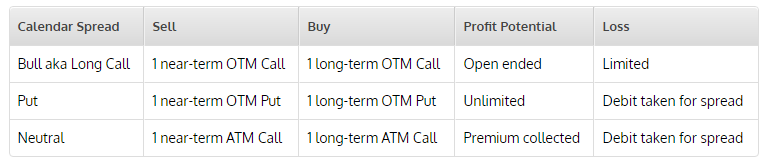### Construction Of A Calendar Spread Strategy

It involves options of:

• an equal number of ATM or slightly OTM calls
• the same underlying stock,
• at the same strike prices, and
• different expiration months

Here, the near month option expires worthless if the price of the underlying at the near month options expiry remains unchanged. The Calendar Spread Strategy would give a payoff resembling this graph: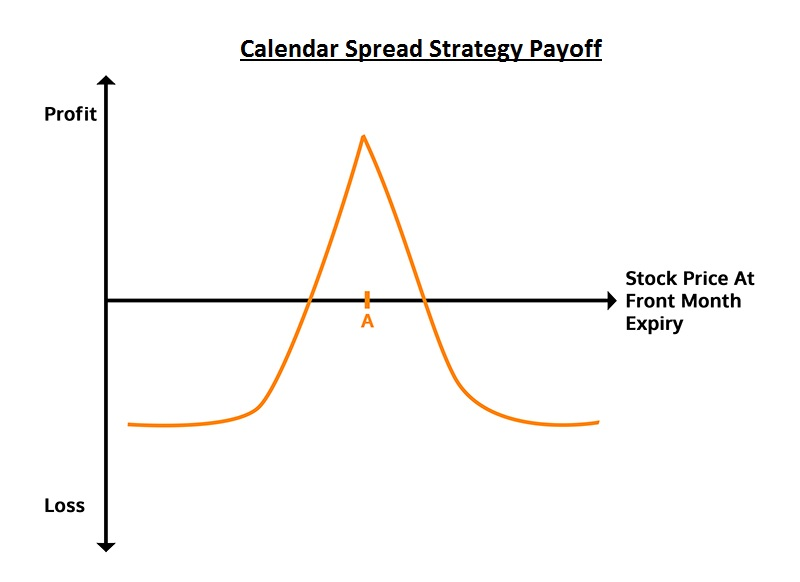A Calendar Spread can be set-up by:

• Selling/short 1 option (front month)
• Buying/long 1 option (back month)
• Both options should be of the same type i.e. either Put or Call
• Both options should have the same strike price
• Based on the same underlying asset

Profit: Ideal Profit is obtained when the short option expires worthless and IV expands in the long option. Max Profit and Breakeven can’t be calculated as both the options have different expiry dates. Potential profit cannot be calculated as the option expire at different times Loss: Max Loss or risk is equal to the initial net debit paid to establish the trade. If the stock price moves dramatically or too far from the strikes, the trade will cause a loss. If all options have the same expiry date, it is indicated by straight lines and sharp angles. Because of the different expiry period of the calls, the lines are not straight.

### Example Of A Calendar Spread

Let's understand the Calendar spread with a simple example of company ABC.

• With ABC stock trading at INR 100.5 in March 2018
• Sell the April 100 call for INR 1.00 (INR 100 for one contract)
• Buy the May 100 call for INR 2.00 (INR 200 for one contract)
• Net cost (debit) INR 1.00 (INR 100 for one contract)

I will use Nifty for this example. The following image captures the month-long movement: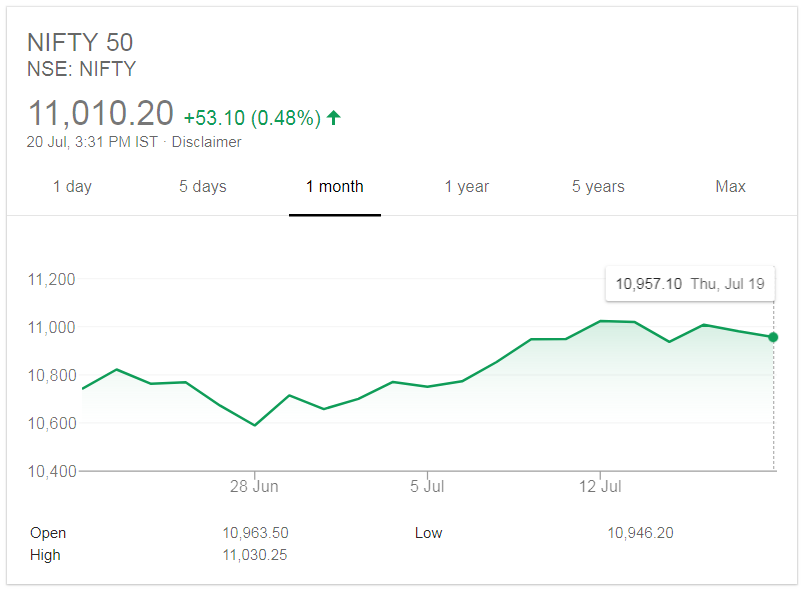Nifty hasn't seen any sudden action in this month so far with the lowest at 10589.10 INR and highest at 11023.20 INR, the highest being just near the present Strike Price of 11010.20 INR. as per Google Finance. As the strategy explains, I will sell 1 call option and buy 1 call option, both At-The-Money (ATM), which in this case is 11023.20 INR. Here's the option chain of Nifty futures for the months of July and August: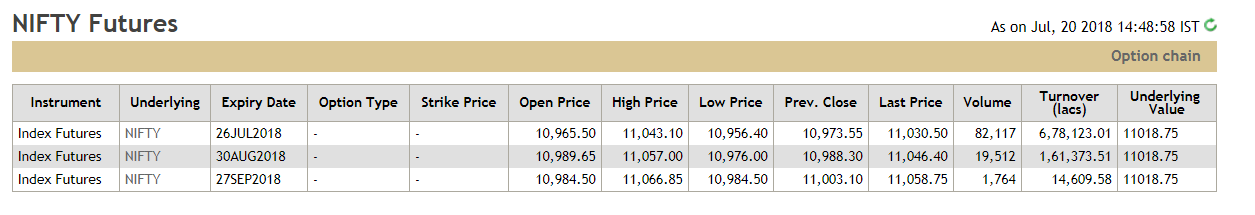Here's the option chain of Nifty for the expiry date of 27th July 2018.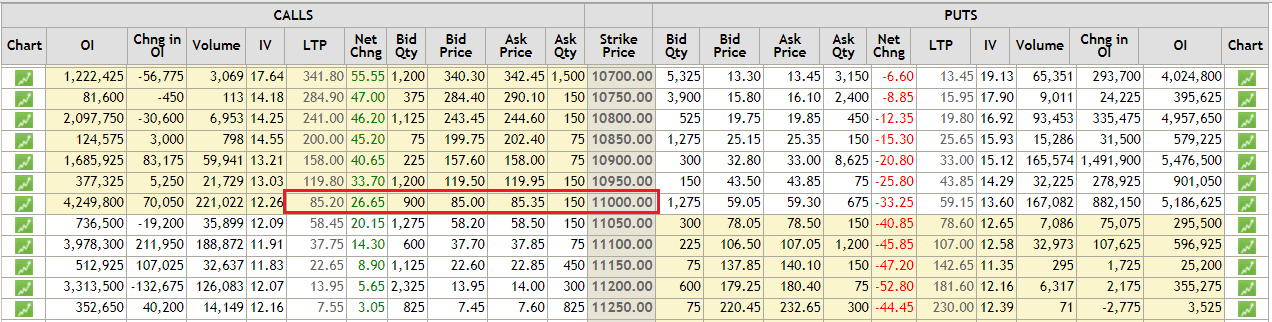Here's the option chain of Nifty for the expiry date of 30th August 2018.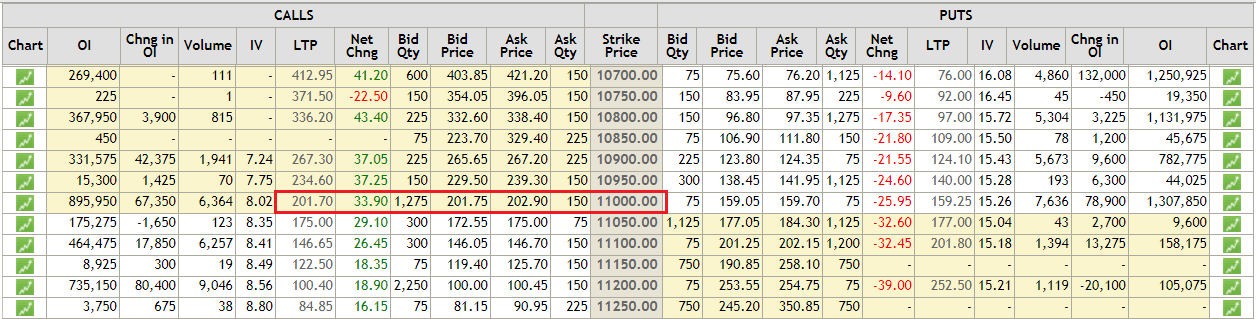Source: nseindia.com

### Calculating The Calendar Spread Payoff

Now, we will go through the Payoff chart using the Python programming code. A Calendar Spread strategy profits from the time decay and/or increase in the implied volatility of the options. In this notebook, we will create a payoff graph of Calendar Spread at the expiry of the front-month option. Importing The Libraries

# Data manipulation
import numpy as np
import pandas as pd

# To plot
import matplotlib.pyplot as plt
import seaborn

# BS Model
import mibian

#### Setup Of A Calendar Spread Strategy

Calendar spread involves options of the same underlying asset, the same strike price but with different expiration dates

• If a Call or Put is Sold with near-term expiration it is called " front-month”
• If a Call or Put is Bought with long-term expiration it is called " back-month”

We will set up the Calendar Spread on Nifty as shown below at the same Strike Price

• Sell 11013.10 strike call @ INR 85.20 expiring on 27 July 2018 — “front-month”
• Buy 11013.10 strike call @ INR 201.70 expiring on 30 August 2018 — “back-month”
# Nifty futures price
nifty_jul_fut = 11030.50
nifty_aug_fut = 11046.40

strike_price = 11013.10
jul_call_price = 85.20
aug_call_price = 201.70
setup_cost = aug_call_price - jul_call_price

# Today's date is 20 July 2018. Therefore, days to July expiry is 7 days and days to August expiry is 41 days.
days_to_expiry_jul_call = 7
days_to_expiry_aug_call = 41

# Range of values for Nifty
sT = np.arange(0.92*nifty_jul_fut,1.1*nifty_aug_fut,1)

#interest rate for input to Black-Scholes model
interest_rate = 0.0


#### Implied Volatility (IV)

We calculate the IV using Black Scholes model for the front-month and back-month call option. To calculate the call price for different values of Nifty, this IV will be used later as an input to the Black-Scholes model.

# Front-month IV
jul_call_iv = mibian.BS([nifty_jul_fut, strike_price, interest_rate, days_to_expiry_jul_call],
callPrice=jul_call_price).impliedVolatility
print ("Front Month IV %.2f" % jul_call_iv,"%")

# Back-month IV
aug_call_iv = mibian.BS([nifty_aug_fut, strike_price, interest_rate, days_to_expiry_aug_call],
callPrice=aug_call_price).impliedVolatility
print ("Back Month IV %.2f" % aug_call_iv,"%")
Front Month IV 12.51 %
Back Month IV 12.52 %

#### Calculating The Call Price For Front And Back Month Option

Since there are two expiration dates for the options in the calendar spread, Black-Scholes pricing model is used to guesstimate the price of the front-month and back-month 11013.10 strike call at the front-month call expiry. You may change the days to expiry below to see how payoff changes.

Note: We have assumed that all other things such as implied volatility and interest rates remain constant.
# Changing days to expiry to a day before the front-month expiry
days_to_expiry_jul_call = 0.001
days_to_expiry_aug_call = 41 - days_to_expiry_jul_call

df = pd.DataFrame()
df['nifty_price'] = sT
df['jul_call_price'] = np.nan
df['aug_call_price'] = np.nan

# Calculating call price for different possible values of Nifty
for i in range(0,len(df)):
df.loc[i,'jul_call_price'] = mibian.BS([df.iloc[i]['nifty_price'], strike_price, interest_rate, days_to_expiry_jul_call],
volatility=jul_call_iv).callPrice

# Since, interest rate is considered 0%, 35 is added to the nifty price to get the Nifty August futures price.
df.loc[i,'aug_call_price'] = mibian.BS([df.iloc[i]['nifty_price']+35, strike_price, interest_rate, days_to_expiry_aug_call],
volatility=aug_call_iv).callPrice
df.head()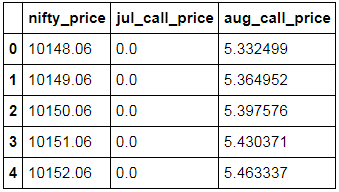df['payoff'] = df.aug_call_price - df.jul_call_price - setup_cost
plt.figure(figsize=(10,5))
plt.ylabel("payoff")
plt.xlabel("Nifty Price")
plt.plot(sT,df.payoff)
plt.show()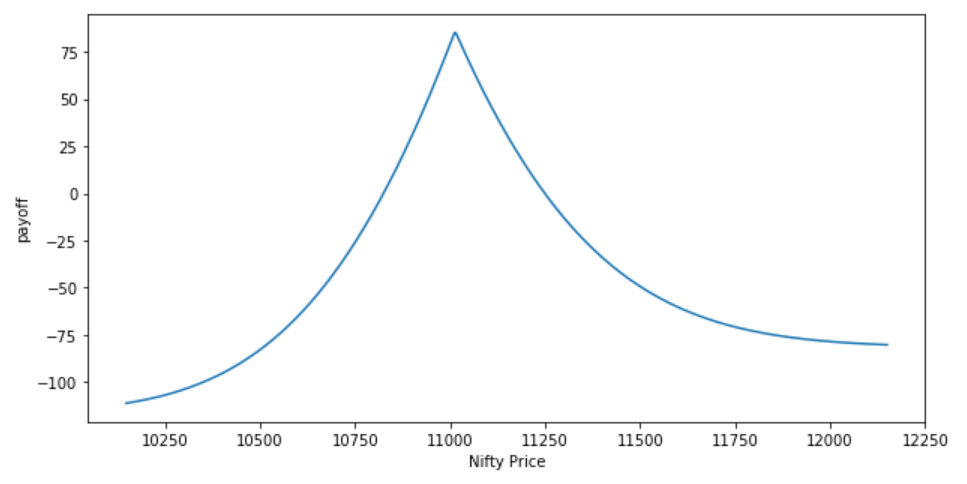#### Graph Interpretation

Max. Profit: When the Nifty price (on expiry of front-month) is at the strike price of INR 11013.10. It decreases when we move in either direction away from the Strike Price. Max. Loss: When the option moves deep ITM (In-The-Money) or deep OTM (Out-of-The-Money).

### Conclusion

• Expecting minimum movement of the stock → Use ATM Calls → Construct Calendar Spread
• Slightly Bullish → Use OTM Calls → Gives Lower up-front Cost

In this strategy, people expect minimal movement of the stock but within a stipulated period of time. This strategy is beneficial to successful, experienced traders and seasoned veterans as it adds profit to their portfolio. You can also refer to this Quora link for information.

You can enroll for the options trading course on Quantra to create successful strategies and implement knowledge in your trading. It covers both retail and institutional trading strategies.